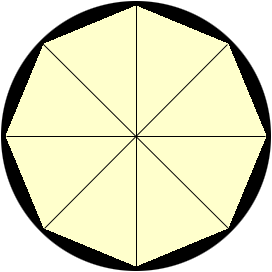SEARCH HOMEMath Central Quandaries & QueriesSubject: Math Question (Pre-Calc) Name: Katy Who are you: Student I have to provide a written description to the following: If a regular hexagon is inscribed in a circle of radius 6.72 centimeters, find the length of one side of the pentagon. How would I got about explaining this? Thanks a bunch. KatyHi Katy,

You said hexagon and then in the same sentence pentagon and I wasn't sure which you wanted so I decided to use an octagon.

Inscribe a regular octagon in a circle. Join each vertex of the octagon to the centre of the circle.The octagon is thus partitioned into eight isosceles triangles, each with a central angle of measure 360/8 = 45 degrees and two sides of length 6.72 centimeters. Can you find the length of the third side?

PennyMath Central is supported by the University of Regina and The Pacific Institute for the Mathematical Sciences.